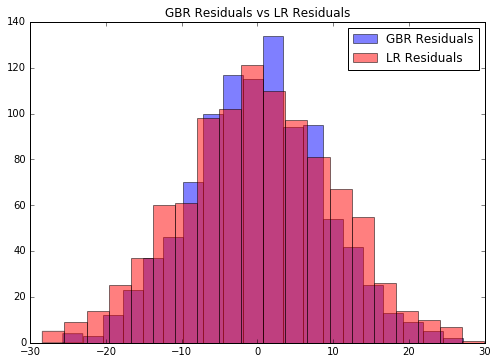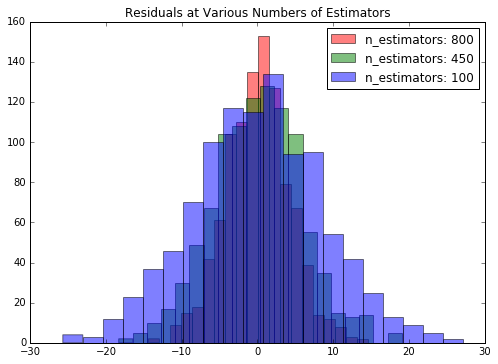# 背景

- 每个学习算法准备率都不高，但是它们集成起来可以获得很好的准确率。
- 这些学习算法依次应用，也就是说每个学习算法都是在前一个学习算法的错误中学习

# 准备模拟数据

import numpy as np
from sklearn.datasets import make_regression
X, y = make_regression(1000, 2, noise=10)

# GBR原理

GBR算是一种集成模型因为它是一个集成学习算法。这种称谓的含义是指GBR用许多较差的学习算法组成了一个更强大的学习算法：

from sklearn.ensemble import GradientBoostingRegressor as GBR
gbr = GBR()
gbr.fit(X, y)
gbr_preds = gbr.predict(X)

from sklearn.linear_model import LinearRegression
lr = LinearRegression()
lr.fit(X, y)
lr_preds = lr.predict(X)

gbr_residuals = y - gbr_preds
lr_residuals = y - lr_preds
%matplotlib inline
from matplotlib import pyplot as plt
f, ax = plt.subplots(figsize=(7, 5))
f.tight_layout()
ax.hist(gbr_residuals,bins=20,label='GBR Residuals', color='b', alpha=.5);
ax.hist(lr_residuals,bins=20,label='LR Residuals', color='r', alpha=.5);
ax.set_title("GBR Residuals vs LR Residuals")
ax.legend(loc='best');np.percentile(gbr_residuals, [2.5, 97.5])
array([-17.14322801,  17.05182403])

np.percentile(lr_residuals, [2.5, 97.5])
array([-19.79519628,  20.09744884])


GBR的置信区间更小，数据更集中，因此其拟合效果更好；我们还可以对GBR算法进行一些调整来改善效果。我用下面的例子演示一下，然后在下一节介绍优化方法：

n_estimators = np.arange(100, 1100, 350)
gbrs = [GBR(n_estimators=n_estimator) for n_estimator in n_estimators]
residuals = {}
for i, gbr in enumerate(gbrs):
gbr.fit(X, y)
residuals[gbr.n_estimators] = y - gbr.predict(X)
f, ax = plt.subplots(figsize=(7, 5))
f.tight_layout()
colors = {800:'r', 450:'g', 100:'b'}
for k, v in residuals.items():
ax.hist(v,bins=20,label='n_estimators: %d' % k, color=colors[k], alpha=.5);
ax.set_title("Residuals at Various Numbers of Estimators")
ax.legend(loc='best');# GBR参数设置

loss参数决定损失函数，也直接影响误差。ls是默认值，表示最小二乘法（least squares）。还有最小绝对值差值，Huber损失和分位数损失（quantiles）等等。

03-27173711-2847
02-0238
02-254728
01-099687
12-22900
08-032608
08-14172
01-29576
03-161万+